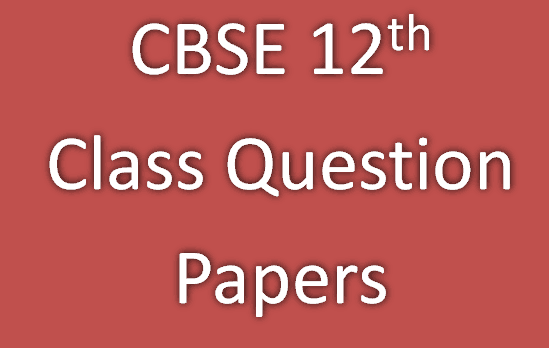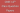# Cbse class 12 2018 question papersHello Guys,

Cbse class 12, 2018 exam is already finished. This year exam pattern was the same as in previous years. This year also cbse provide different sets of question papers of all subjects. All sets have almost the same level of question. So Your waiting is over. Here we are updating CBSE class 12 2018 question papers of different subjects. Here we are attaching the marking scheme of all the subjects too.## Cbse class 12 2018 question papers – Maths

This year CBSE provide 3 sets of question papers. The question paper consists of 29 questions divided into four sections A, B, C, and D.

• A comprises of 4 questions of one mark each.
• Section B contains 8 questions of two marks each.
• C comprises of 11 questions of four marks each.
• Section D contains 6 questions of six marks each.

65-1 Maths
65-2 Maths
65-3 Maths
Marking Scheme

## CBSE question paper class 12 – Physics

This year CBSE provide 3 sets of question papers. There are 26 questions in all. This question paper has five sections: Section A, Section B, Section C, Section D, and Section E.

• A comprises five questions of one mark each.
• B contains five questions of two marks each.
• C comprises twelve questions of three marks each.
• D contains one value based question of four marks.
• E comprises three questions of five marks each.

55-1 Physics
55-2 Physics
55-3 Physics
Marking Scheme

## cbse question paper class 12 – Chemistry

• Questions 1 to 5 are very short answer questions and carry 1 mark each.
• Questions number 6 to 10 are short answer questions and carry 2 marks each.
• 11 to 22 are also short answer questions and carry 3 marks each.
• Question number 23 is a value based question and carries 4 marks.
• Questions number 24 to 26 are long answer questions and carry 5 marks each.

56-1 Chemistry
56-2 Chemistry
56-3 Chemistry
Marking Scheme

Read More – Jee Mains 2018

## Cbse class 12 2018 question papers – Biology

• There are a total of 26 questions and five sections in the question paper.
• A contains questions number 1 to 5, very short-answer type questions of 1 mark each.
• B contains questions number 6 to 10, short-answer type I questions of 2 marks each.
• C contains questions number 11 to 22, also short-answer type II questions of 3 marks each.
• D contains question number 23, value based question of 4 marks.
• E contains questions number 24 to 26, long-answer type questions of 5 marks each.